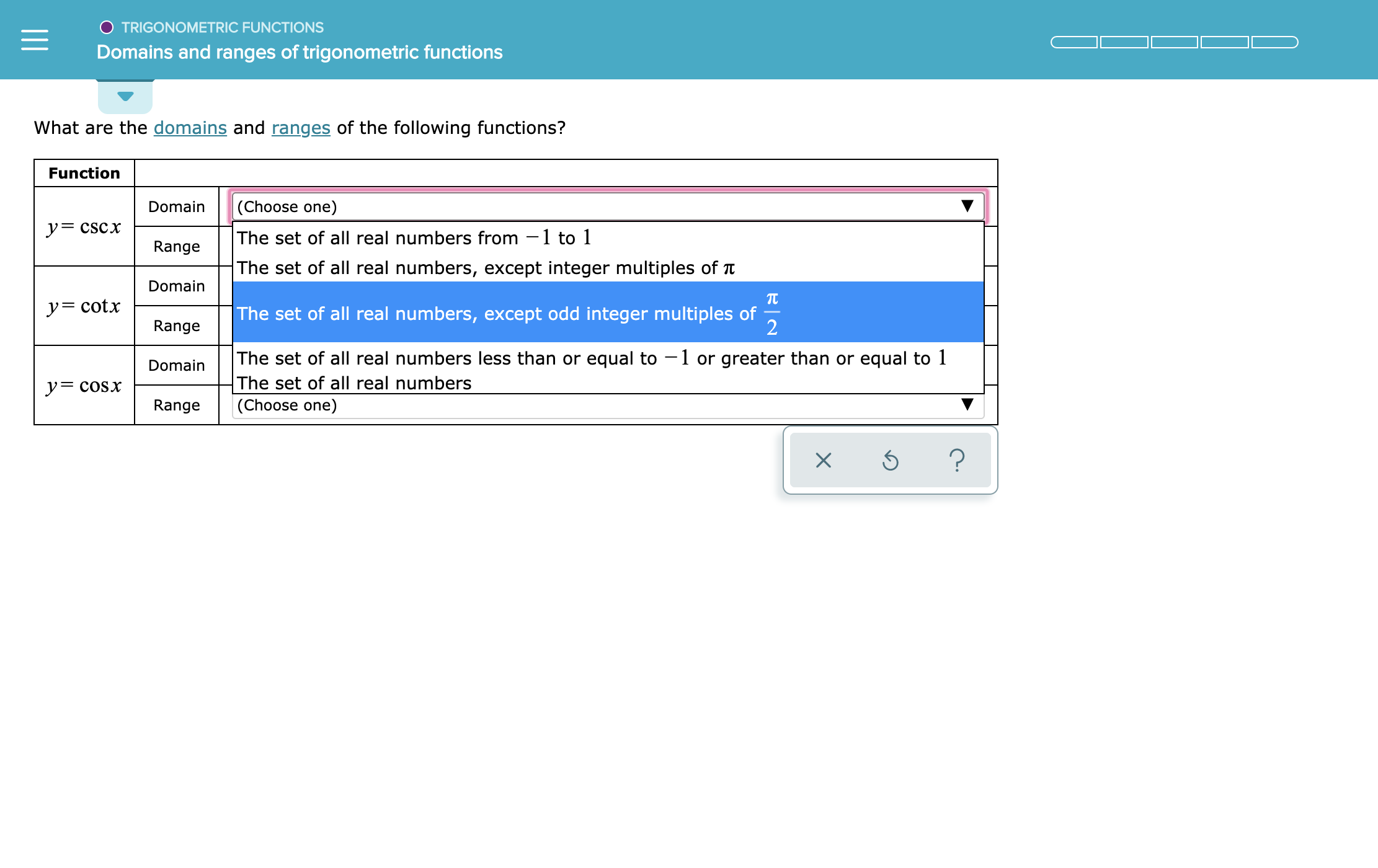O TRIGONOMETRIC FUNCTIONSDomains and ranges of trigonometric functionsWhat are the domains and ranges of the following functions?Function(Choose one)DomainyCSCxThe set of all real numberss from -1 to 1RangeThe set of all real numbers, except integer multiples of tDomainy= cotxThe set of all real numbers, except odd integer multiples of2RangeThe set of all real numbers less than or equal to 1 or greater than or equal to 1The set of all real numbers(Choose one)Domainy=coSxRangeX

Question

see attachmenthelp_outlineImage TranscriptioncloseO TRIGONOMETRIC FUNCTIONS Domains and ranges of trigonometric functions What are the domains and ranges of the following functions? Function (Choose one) Domain yCSCx The set of all real numberss from -1 to 1 Range The set of all real numbers, except integer multiples of t Domain y= cotx The set of all real numbers, except odd integer multiples of 2 Range The set of all real numbers less than or equal to 1 or greater than or equal to 1 The set of all real numbers (Choose one) Domain y=coSx Range X fullscreen
Step 1

Calculation:

Compute the domain and the range of the function  y = csc(x)  as follows.

Rewrite y = csc(x) as y = 1/sin(x).

since sin(0) = 0, the function is undefined for all integer multiplies of π and for 0.

Thus, the domain of y = csc(x) is the set of all real numbers expect the integer multiplies of π.

Range of y = csc(x) is set of all real numbers less than or equal to −1 or greater than or equal to 1...

Want to see the full answer?

See Solution

Want to see this answer and more?

Our solutions are written by experts, many with advanced degrees, and available 24/7

See Solution
Tagged in

Other